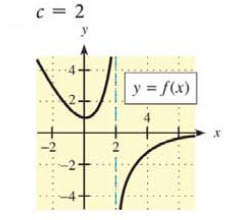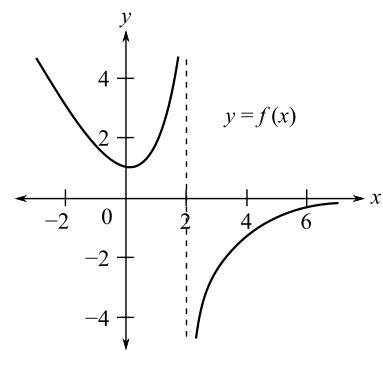Chapter 9.1, Problem 7EMathematical Applications for the ...

12th Edition
Ronald J. Harshbarger + 1 other
ISBN: 9781337625340

Solutions

Chapter
SectionMathematical Applications for the ...

12th Edition
Ronald J. Harshbarger + 1 other
ISBN: 9781337625340
Textbook Problem

In Problems 7-10, use the graph of y = f ( x ) and the given c-value to find the following, whenever they exist.(a) lim x → c − f ( x ) (b) lim x → c + f ( x ) (c) lim x → c f ( x ) (d) f ( c ) c = 2(a)

To determine

The value of limxcf(x) by the use of the graph,Explanation

Given Information:

The value of c is 2. The graph of y=f(x) is

Explanation:

Consider the provided graph,

The value of c is equal to 2.

The limit from the left is represented by limx2f(x), that is, the values of

(b)

To determine

The value of limxc+f(x) by the use of the graph,(c)

To determine

The value of limxcf(x) by the use of the graph,(d)

To determine

The value of f(c) by the use of the graph,Still sussing out bartleby?

Check out a sample textbook solution.

See a sample solution

The Solution to Your Study Problems

Bartleby provides explanations to thousands of textbook problems written by our experts, many with advanced degrees!

Get Started

Find all possible real solutions of each equation in Exercises 3144. x31=0

Finite Mathematics and Applied Calculus (MindTap Course List)

Calculate y'. 20. y = exsec x

Single Variable Calculus: Early Transcendentals, Volume I

Evaluate the integral. 13. x29x3dx

Calculus: Early Transcendentals